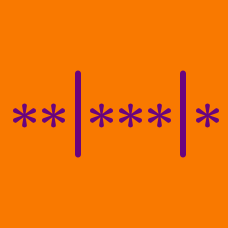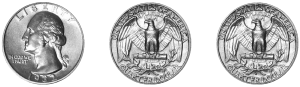Probability

Objects Into Bins WarmupIf 10 people each flip a coin 3 times, is it possible for each of the 10 people to get a different sequence of Heads and Tails?If 10 people each divide 4 balls — colored blue, green, orange, and purple — into 2 groups, is it possible for each of the 10 people to choose a different division?

Note: Each group must contain at least one ball. The groups are not labeled; a group is determined only by the balls it contains. Two divisions are considered different if there is a pair of balls that are both in the same group in one division but are in different groups in the other.

$6 = 6, \quad 6 = 4 + 1 + 1, \quad 6 = 3 + 3, \quad \ldots$ If 10 people each write 6 as a sum of non-increasing positive integers, is it possible for each of the 10 people to choose a different sum?

Note: The single number 6 is considered to be a sum, even though there is no $+$ sign.

A sum of non-increasing positive integers is a sum whose terms do not increase from left to right (though they may remain constant). For example, $6 = 4 + 1 + 1$ is a sum of non-increasing positive integers, since $4 > 1 = 1,$ but $1 + 4 + 1$ is not, since $1 < 4.$

$202, 31, 4, 112, \ldots$

If 15 people each write down a positive integer with fewer than 4 digits whose digits add up to 4, is it possible for each of the 15 people to write a different number?

4 friends visit an ice cream parlor that offers vanilla, chocolate, and strawberry ice cream. How many ways are there for the 4 friends to each order a different flavor of ice cream?

Note: Assume each person only orders a single flavor.

×